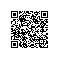# 烦恼# 环境（注：样例数据来自于匿名化处理后的真实数据集，下载自superdatascience官网。）

jupyter notebook# 清理

import numpy as npimport pandas as pd

customer_churn.csv里读入数据：• RowNumber：行号，这个肯定没用，删除
• CustomerID：用户编号，这个是顺序发放的，删除
• Surname：用户姓名，对流失没有影响，删除
• CreditScore：信用分数，这个很重要，保留
• Geography：用户所在国家/地区，这个有影响，保留
• Gender：用户性别，可能有影响，保留
• Age：年龄，影响很大，年轻人更容易切换银行，保留
• Tenure：当了本银行多少年用户，很重要，保留
• Balance：存贷款情况，很重要，保留
• NumOfProducts：使用产品数量，很重要，保留
• HasCrCard：是否有本行信用卡，很重要，保留
• IsActiveMember：是否活跃用户，很重要，保留
• EstimatedSalary：估计收入，很重要，保留
• Exited：是否已流失，这将作为我们的标签数据

X = df.loc[:,['CreditScore', 'Geography', 'Gender', 'Age', 'Tenure', 'Balance', 'NumOfProducts', 'HasCrCard', 'IsActiveMember', 'EstimatedSalary']]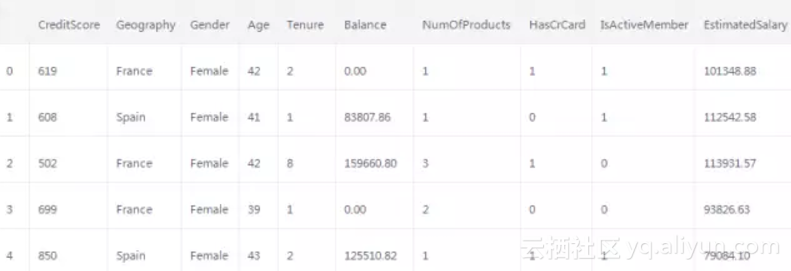y = df.Exited

0 11 02 13 04 0Name: Exited, dtype: int64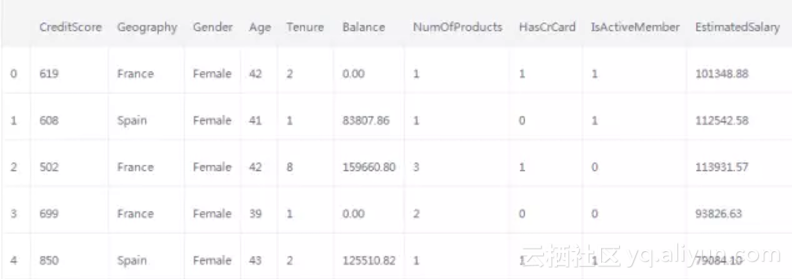from sklearn.preprocessing import LabelEncoder, OneHotEncoderlabelencoder1 = LabelEncoder()X.Geography= labelencoder1.fit_transform(X.Geography)labelencoder2 = LabelEncoder()X.Gender = labelencoder2.fit_transform(X.Gender)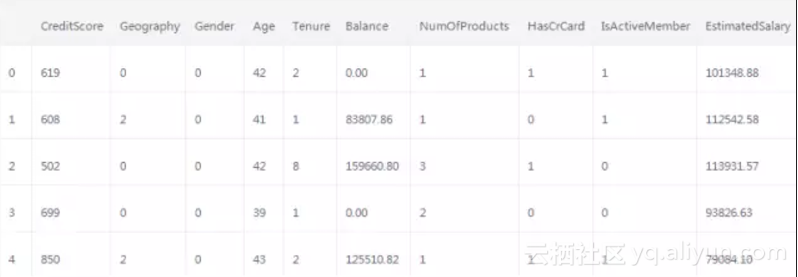onehotencoder = OneHotEncoder(categorical_features = )X = onehotencoder.fit_transform(X).toarray()

X

array([ 1.00000000e+00, 0.00000000e+00, 0.00000000e+00, 6.19000000e+02, 0.00000000e+00, 4.20000000e+01, 2.00000000e+00, 0.00000000e+00, 1.00000000e+00, 1.00000000e+00, 1.00000000e+00, 1.01348880e+05])

X = np.delete(X, , 1)

X

array([ 0.00000000e+00, 0.00000000e+00, 6.19000000e+02, 0.00000000e+00, 4.20000000e+01, 2.00000000e+00, 0.00000000e+00, 1.00000000e+00, 1.00000000e+00, 1.00000000e+00, 1.01348880e+05])

0 11 02 13 04 0Name: Exited, dtype: int64

y = y[:, np.newaxis]y

array([, , , ..., , , ])

onehotencoder = OneHotEncoder()y = onehotencoder.fit_transform(y).toarray()

y

array([[ 0., 1.], [ 1., 0.], [ 0., 1.], ..., [ 0., 1.], [ 0., 1.], [ 1., 0.]])

from sklearn.model_selection import train_test_splitX_train, X_test, y_train, y_test = train_test_split(X, y, test_size = 0.2, random_state = 0)

len(X_train)

8000

len(X_test)

2000

from sklearn.preprocessing import StandardScalersc = StandardScaler()X_train = sc.fit_transform(X_train)X_test = sc.transform(X_test)

X_train

array([[-0.5698444 , 1.74309049, 0.16958176, ..., 0.64259497, -1.03227043, 1.10643166], [ 1.75486502, -0.57369368, -2.30455945, ..., 0.64259497, 0.9687384 , -0.74866447], [-0.5698444 , -0.57369368, -1.19119591, ..., 0.64259497, -1.03227043, 1.48533467], ..., [-0.5698444 , -0.57369368, 0.9015152 , ..., 0.64259497, -1.03227043, 1.41231994], [-0.5698444 , 1.74309049, -0.62420521, ..., 0.64259497, 0.9687384 , 0.84432121], [ 1.75486502, -0.57369368, -0.28401079, ..., 0.64259497, -1.03227043, 0.32472465]])

# 决策树

from sklearn import treeclf = tree.DecisionTreeClassifier()clf = clf.fit(X_train, y_train)

y_pred = clf.predict(X_test)

y_pred

array([[ 1., 0.], [ 0., 1.], [ 1., 0.], ..., [ 1., 0.], [ 1., 0.], [ 0., 1.]])

from sklearn.metrics import classification_reportprint(classification_report(y_test, y_pred))

precision recall f1-score support 0 0.89 0.86 0.87 1595 1 0.51 0.58 0.54 405avg / total 0.81 0.80 0.81 2000

# 深度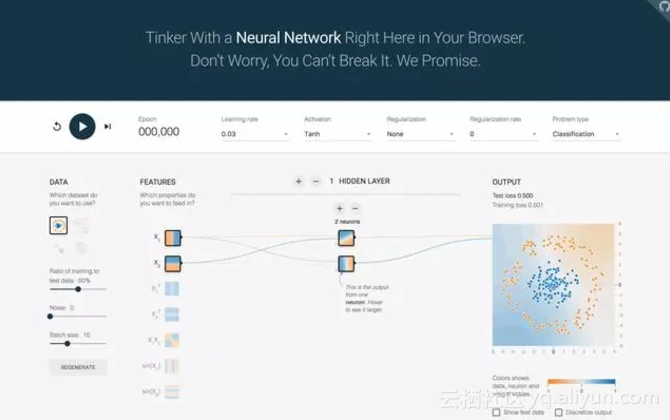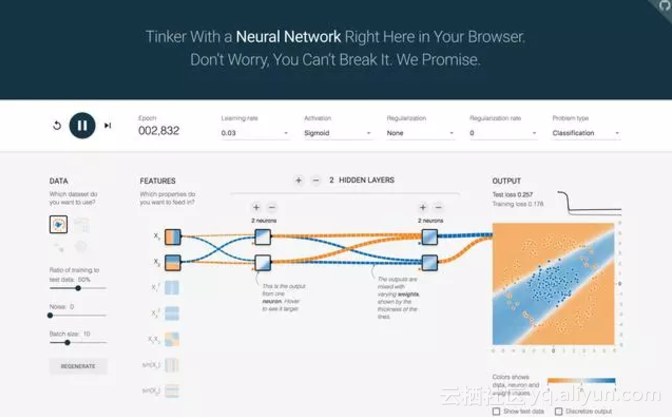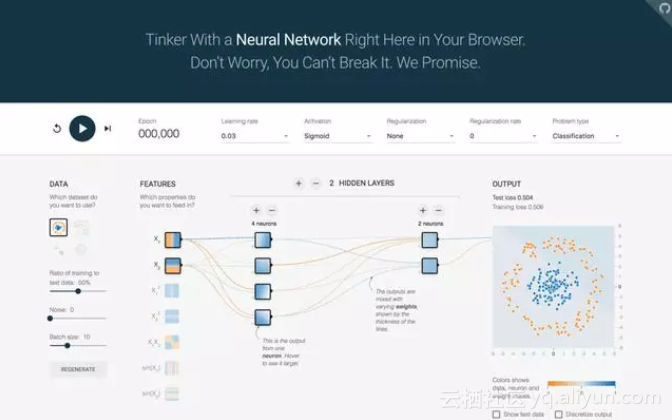# 实战

pip install tensorflowpip install tflearn

import tflearn

net = tflearn.input_data(shape=[None, 11])

shape的第一项，None，指的是我们要输入的特征矩阵行数。因为我们现在是搭建模型，后面特征矩阵有可能一次输入，有可能分成组块输入，长度可大可小，无法事先确定。所以这里填None。tflearn会在我们实际执行训练的时候，自己读入特征矩阵的尺寸，来处理这个数值。

net = tflearn.fully_connected(net, 6, activation='relu')net = tflearn.fully_connected(net, 6, activation='relu')net = tflearn.fully_connected(net, 6, activation='relu')

activation刚才在深度学习游乐场里面我们遇到过，代表激活函数。如果没有它，所有的输入输出都是线性关系。

Relu函数是激活函数的一种。它大概长这个样子。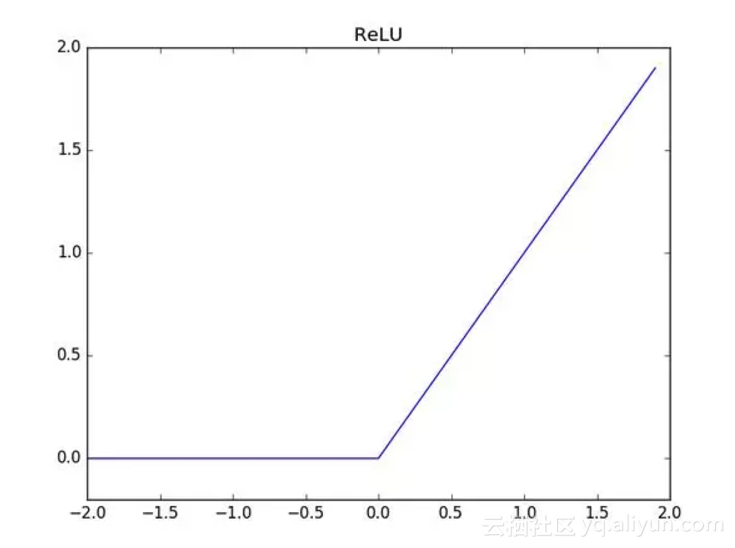net = tflearn.fully_connected(net, 2, activation='softmax')net = tflearn.regression(net)

model = tflearn.DNN(net)

model.fit(X_train, y_train, n_epoch=30, batch_size=32, show_metric=True)

 n_epoch：数据训练几个轮次。batch_size：每一次输入给模型的数据行数。show_metric：训练过程中要不要打印结果。

Training Step: 7499 | total loss: [1m[32m0.39757[0m[0m | time: 0.656s| Adam | epoch: 030 | loss: 0.39757 - acc: 0.8493 -- iter: 7968/8000Training Step: 7500 | total loss: [1m[32m0.40385[0m[0m | time: 0.659s| Adam | epoch: 030 | loss: 0.40385 - acc: 0.8487 -- iter: 8000/8000--

tensorboard --logdir=/tmp/tflearn_logs/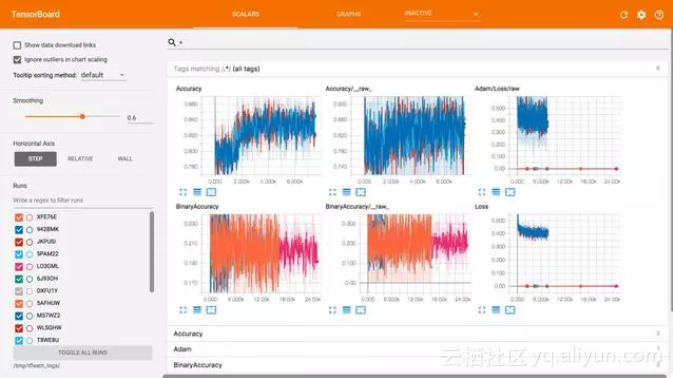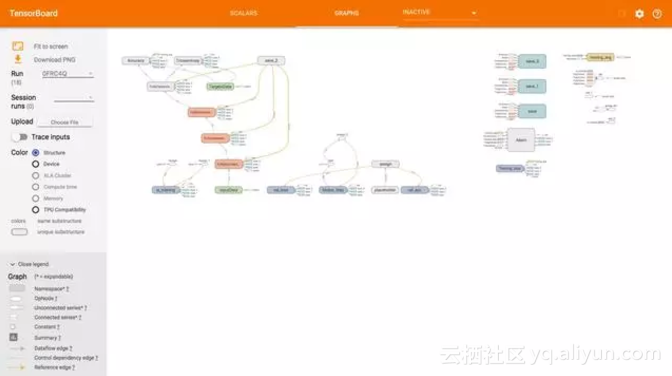# 评估

X_test

array([ 1.75486502, -0.57369368, -0.55204276, -1.09168714, -0.36890377, 1.04473698, 0.8793029 , -0.92159124, 0.64259497, 0.9687384 , 1.61085707])

y_pred = model.predict(X_test)

y_pred

array([ 0.70956731, 0.29043278], dtype=float32)

y_test

array([ 1., 0.])

score = model.evaluate(X_test, y_test)print('Test accuarcy: %0.4f%%' % (score * 100))

Test accuarcy: 84.1500%

# 说明